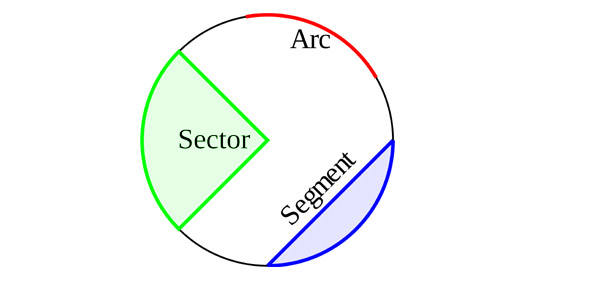# Finding The Circumference And Diameter Of A Circle

9 Questions | Attempts: 3303
ShareSettingsThis quiz was created to demonstrate understanding of finding the radius, diameter, and circumference of a circle.

• 1.
1.  Sally purchased a CD that was 5 inches in diameter.  What is the circumference of the CD?
• A.

25 inches

• B.

15.7 inches

• C.

14 inches

• D.

20 inches

• 2.
The basketball team plays with a ball with a radius of  6 inches.  What is the circumference of each basketball?
• A.

36.68 inches

• B.

37.68 inches

• C.

38.68 inches

• D.

39.68 inches

• 3.
Nathan and three of his friends were building a duck blind to go hunting.  They knew for all of them to fit inside that they needed a circumference of 15.7 yards.  What diameter must the blind have for it to have the correct circumference?
• A.

3 yards

• B.

4 yards

• C.

5 yards

• D.

6 yards

• 4.
If the diameter of a circle is 15 cm, what is the circumference of the circle?
• A.

45.1 cm

• B.

46.1 cm

• C.

47.1 cm

• D.

48.1 cm

• 5.
When measuring the hula hoop in class, group C discovered that it had a radius of 7 inches.  What is the circumference of the hula hoop?
• A.

39.6 inches

• B.

37.6 inches

• C.

47 .6 inches

• D.

49.6 inches

• 6.
The laundry basket that Mrs. Smith uses for recycling has a diameter of 25 cm.  She wants to move the basket to another place in her classroom.  In order for her to complete this task, she must know the circumference of the basket.  Please find teh circumference of the basket so Mrs. Smith can determine where to place it.
• A.

76.5 cm

• B.

77.5 cm

• C.

78.5 cm

• D.

79.5 cm

• 7.
When determine the circumference of a circle, you must find the ____________ and multiply it by 3.14.
• A.

Area

• B.

• C.

Circumference

• D.

Diameter

• 8.
The radius of a circle tells
• A.

The distance around the outside of the circle.

• B.

The distance across the whole circle.

• C.

The distance from the midpoint around the outside.

• D.

The distance from the midpoint to one side of the circle.

• 9.
The table we have to sit at has a circumference of 6.28 yards.  We need to find the diameter of the table.
• A.

1 yard

• B.

2 yards

• C.

3 yards

• D.

4 yards

## Related TopicsBack to top
×

Wait!
Here's an interesting quiz for you.